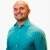# SAT Prep Learn how to use proportionsThis lesson will look at an example problem involving proportions. This lesson will give an explanation of how to use proportions to determine the number of pears used in a salad with a step by step... This lesson will look at an example problem involving proportions. This lesson will give an explanation of how to use proportions to determine the number of pears used in a salad with a step by step look to better explain the concept.
More... Collapse
4 Views